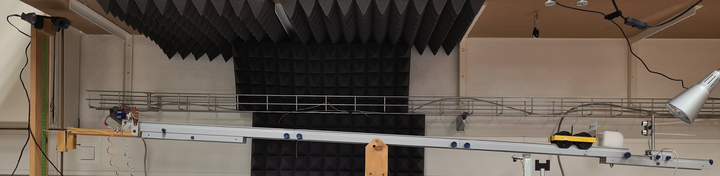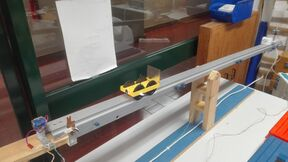# Inclined Plane

## Experiment description

The inclined plane is one of the six classic simple machines. Inclined planes are generally used to move heavy loads over vertical obstacles, such as ramps to move cargo.

Moving an object up on an inclined plane requires less force than lifting it vertically, as if we reduced gravity! This mechanical advantage, by which the force is reduced, is equal to the ratio between the length of the inclined surface and the height of the plane.

In laboratory experiments that recreate this machine, when an air chute is not used in order to almost eliminate the rolling friction, the plane must have a significant slope so that the gravitational acceleration can be much higher than the friction, making it impossible for this experience to be carried out in a long time, perceptible to human senses.

However, friction is an integral part of the mechanics of the problem. With this experiment, it is possible to determine the friction function through a multivariate analysis, adjusting a function that depends on the inclination of the rail. For this purpose, the experiment allows changing the descent angle and, through this, separating the frictional forces from the gravitational force, obtaining a value very close to 9,8 ms-2.

• Video: rtsp://elabmc.ist.utl.pt/plinc.sdp
• Laboratory: Básico
• Control Room: Inclined Plane
• Level: *

Purposely, this experiment uses a car equipped with a frontal windshield in order to exacerbate the effect of friction and demonstrate that its equation can be determined by multivariate analysis of several rides, for different inclinations.Inclined plane experience assembly where is easy to identify the necessary acoustic insulation to avoid spurious echoes that difficult the detection of the position by the sonar.

### Experimental apparatusGeneral view of the experiment with the sonar at one end, after the collision collector spring.

The inclined plane of this experiment recreates this "reduction of gravity". It consists of a vehicle that moves on a track with adjustable inclination and that tilts at its midpoint. The height of the chute is measured at 1003 mm from the axis of the experiment.

Initially the chute tilts to a position with a negative angle in order to collect and park the car at the origin, about 1.3 m from the spring that will absorb the energy of its movement. The electromagnet immobilizes the car and, subsequently, the rail is raised to the pre-selected height. When it reaches that point, the electromagnet releases the car and it moves freely on the rail until it hits the spring. An ultrasonic detector then collects position samples as a function of the elapsed time, allowing to trace the vehicle's trajectory during the fall and in its final damping.

## Protocol

Based on the pre-selected data in the experiment, obtain a graph of the position as a function of the elapsed time. Based on this data, determine the speed and acceleration graphs. Compare the acceleration obtained value with gravity.

#### Determination of speed as a function of distance traveled

Based on the previous data, determine the speed as a function of the distance traveled eliminating the time in the previous graphs, that is, tracing the curve drawn by the speed pairs, distance for each available time.

#### Determination of the spring restitution constant

Depending on the various parables obtained in the vehicle's damping, determine the relative energy loss in each collision with the spring and determine the spring restitution constant.

As can be quickly inferred, the adjustment of the parabolic model to the movement produces a deviation that is only possible to understand with the inclusion of a friction term. In effect, the car has a front flap designed to induce a certain aerodynamic friction. By adapting the equations to include a rolling friction term (linear with velocity) and aerodynamic (Cx, quadratic dependence with velocity), a more accurate value for the acceleration of the vehicle can finally be determined. Usually the Cx only significantly influences the movement after 25 km/h.

The adjustment of a friction function for the acceleration in the form a = b + c*v + d*v2 allows to extract the local acceleration of gravity when using several sets of data for various angles.

#### Multivariate model

The multivariate analysis used serves to build a model of numerical adjustment for various angles to the various characteristics of the movement obtained. The variation of the angle makes it possible to distinguish the effect of gravity from the effect of rolling friction force, since this is considered independent of the angle. Thus, by making a a=b+c*v+d*v2 type adjustment, the parameter b which implicitly deters the gravity and friction of the bearing (related to the mechanics of the vehicle) can be solved by separating it into b =g*sin(θ)+b friction . The remaining parameters result from a model of the dependence of friction with speed. For high values of this (typically greater than 7-10 ms-1), friction has a strong quadratic dependence on speed - aerodynamic friction - but, in our case, we also have to consider the dependence on mechanical friction (batrito+c*v), since the car departs from rest.

When performing this multivariate analysis, we are considering an independent association between the parameters, a necessary condition for these parameters to be part of a numerical model that will prove correct by the outcome of the adjustment. For this purpose, a numerical solver should be used, interactively adjusting the various covariates, such as that of the MSExcel.

#### Strict determination of the spring restitution constant

A brief analysis makes it possible to conclude that the spring restitution constant for the various strikes is affected by an appreciable error, which increases with the distance traveled, since the work carried out by the frictional force affects the calculation of the mechanical energy in each section of the movement. However, by calculating the work performed by the frictional force based on the frictional force equation determined by the previously described process, it is possible to correctly infer the mechanical energy before and after each impact, allowing to correctly calculate the spring restitution constant.

## Construction details

The most curious component of this experience is the electromagnet who keeps the car safe in the launch position. This electromagnet can be built by wrapping enameled wire over a soft iron core or using an electromagnet extracted from a simple toaster!

Another innovation is the fact that the assembly is carried out on a tilting chute that simplifies the return of the car to the launch position. As it is necessary a reasonable inclination to overcome static friction, the impact on the fixing electromagnet is significant but can be resolved by placing a self-adhesive foam on both sides, between the mooring iron and the car.